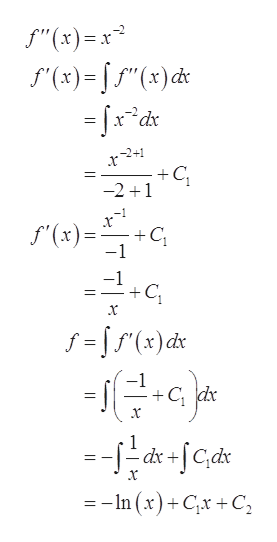# Find f.f''(x) = x-2 , when x is greater than zero. f(1) = 0 , f(2) = 0 .

Question
2 views

Find f.

f''(x) = x-2 , when x is greater than zero. f(1) = 0 , f(2) = 0 .

check_circle

Step 1

To determine the function f(x)

Step 2

Given:

Step 3

Integrate the function twice with...help_outlineImage Transcriptionclose"(x) '(x)()d -xdx +C -2 1 f(x) C -1 -1 +C x f fr)dx -1 +C dr x -dc dx+ --In (x)+Cx +C fullscreen

### Want to see the full answer?

See Solution

#### Want to see this answer and more?

Solutions are written by subject experts who are available 24/7. Questions are typically answered within 1 hour.*

See Solution
*Response times may vary by subject and question.
Tagged in

### Integration